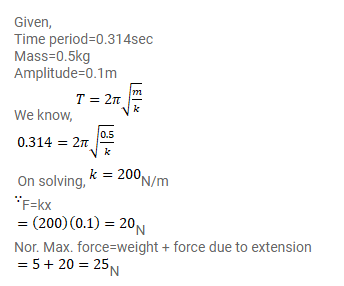# A block of mass 0.5kg hanging from a vertical spring executes simple harmonic

Question:

A block of mass $0.5 \mathrm{~kg}$ hanging from a vertical spring executes simple harmonic motion of amplitude $0.1 \mathrm{~m}$ and time period $0.314 \mathrm{~s}$. Find the maximum force exerted by the spring on the block.

Solution: